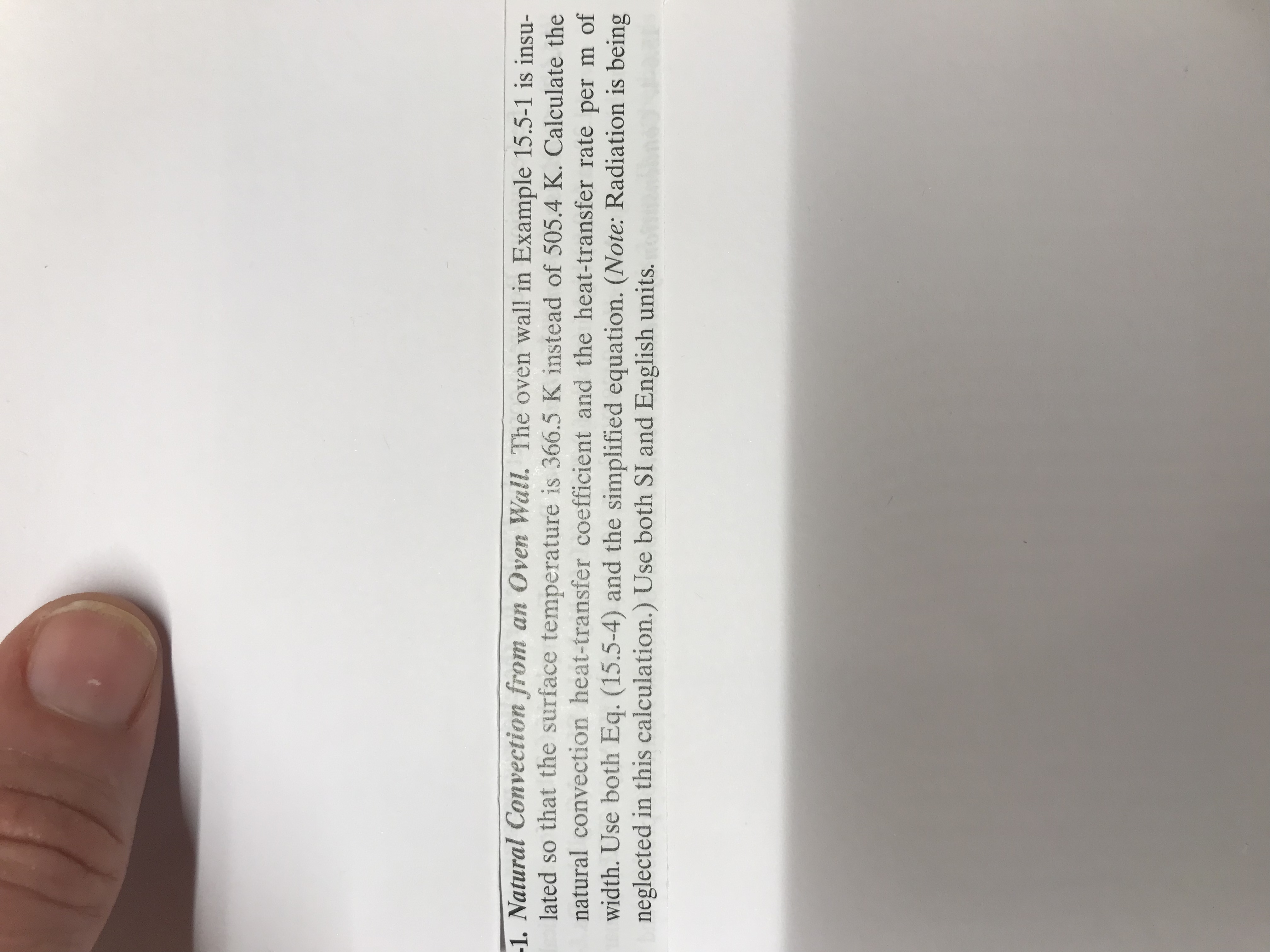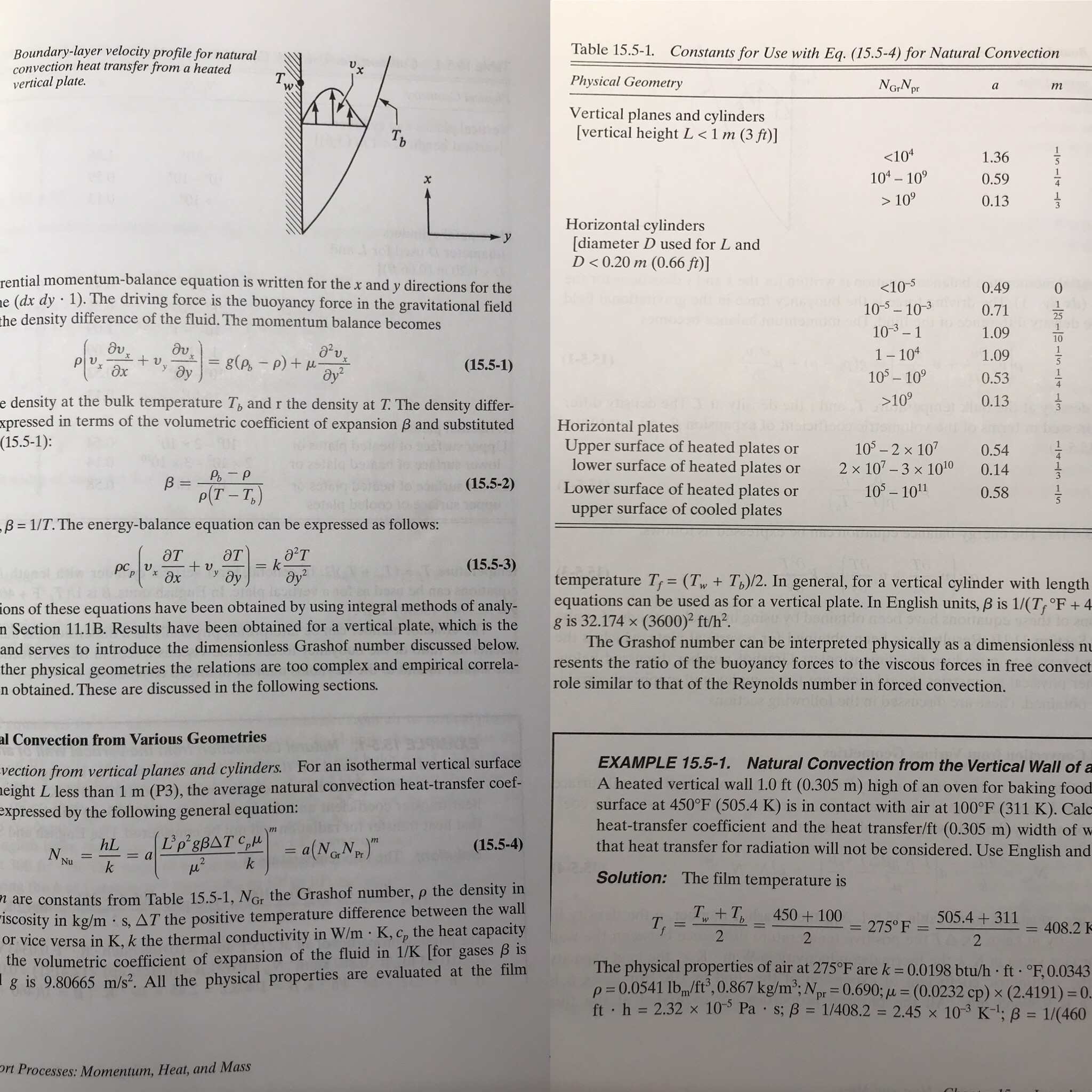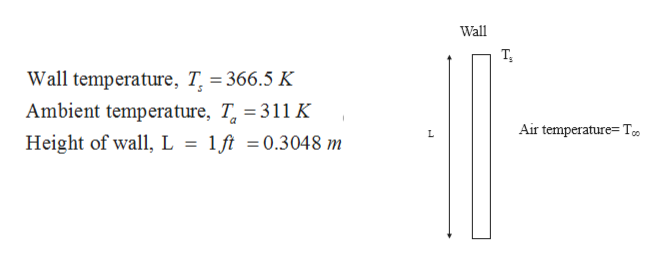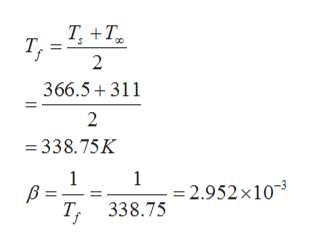# -1. Natural Convection from an Oven Wall. The oven wall in Example 15.5-1 is insu-lated so that the surface temperature is 366.5 K instead of 505.4 K. Calculate thenatural convection heat-transfer coefficient and the heat-transfer rate per m ofwidth. Use both Eq. (15.5-4) and the simplified equation. (Note: Radiation is beingneglected in this calculation.) Use both SI and English units. Table 15.5-1. Constants for Use with Eq. (15.5-4) for Natural ConvectionBoundary-layer velocity profile for naturalconvection heat transfer froma heatedvertical plate4047Physical GeometryNGrNprатVertical planes and cylinders[vertical height L 100.13Horizontal cylinders[diameter D used for L andD1090.13Horizontal platesUpper surface of heated plateslower surface of heated platesLower surface of heated platesupper surface of cooled plates105- 2 x 1072 x 107 -3 x 10100.54or0.14orВ 3p(Т - Т,)(15.5-2)10- 1010.58orB = 1/T. The energy-balance equationcan be expressed as follows:Э'тӘтЭт(15.5-3)k+ v.yрс,ay2дудхtemperature T = (T, + Th)/2. In general, for a vertical cylinder with lengthequationsg is 32.174 x (3600)2 ft/h2.The Grashof number can be interpreted physicallyresents the ratio of the buoyancy forces to the viscous forces in free convectrole similar to that of the Reynolds number in forced convection.can be used as for a vertical plate. In English units, B is 1/(T;°F + 4ions of these equations have been obtained by using integral methods of analy-n Section 11.1B. Results have been obtained for a vertical plate, which is thec bprctas a dimensionless nuand serves to introduce the dimensionless Grashof number discussed below.ther physical geometries the relations are too complex and empirical correla-n obtained. These are discussed in the following sections.al Convection from Various Geometriesvection from vertical planes and cylinders. For an isothermal vertical surfaceeight L less than 1 mexpressed by the following general equation:EXAMPLE 15.5-1. Natural Convection from the Vertical Wall of aA heated vertical wall 1.0 ft (0.305 m) high of an oven for baking foodsurface at 450°F (505.4 K) is in contact with air at 100°F (311 K). Calcheat-transfer coefficient and the heat transfer/ft (0.305 m) width of wthat heat transfer for radiation will not be considered. Use English and(P3), the average natural convection heat-transfer coef-(Ľp'gBAT C, a(NGNphL(15.5-4)NNuPra2kkSolution: The film temperature isare constants from Table 15.5-1, NGr the Grashof number, p the density in-ISCOsity in kg/m s, AT the positive temperature difference between the wallor vice versa in K, k the thermal conductivity in W/m K, c, the heat capacitythe volumetric coefficient of expansion of the fluid in 1/K [for gases B isg is 9.80665 m/s2. All the physical propertiesTy +TT,450+100= 275° F- 505.4 +311408.2f222The physical properties of air at 275°F are k 0.0198 btu/h ft °F,0.0343p 0.0541 lbm/ft', 0.867 kg/m; Npr= 0.690; L = (0.0232 cp) x (2.4191) 0.ft h 2.32 x 10 Pa s; B = 1/408.2 2.45 x 103 K1; B 1/(460are evaluated at the filmrt Processes: Momentum, Heat, and MassO3-1-n-1-1m1n

Question
72 viewshelp_outlineImage Transcriptionclose-1. Natural Convection from an Oven Wall. The oven wall in Example 15.5-1 is insu- lated so that the surface temperature is 366.5 K instead of 505.4 K. Calculate the natural convection heat-transfer coefficient and the heat-transfer rate per m of width. Use both Eq. (15.5-4) and the simplified equation. (Note: Radiation is being neglected in this calculation.) Use both SI and English units. fullscreenhelp_outlineImage TranscriptioncloseTable 15.5-1. Constants for Use with Eq. (15.5-4) for Natural Convection Boundary-layer velocity profile for natural convection heat transfer froma heated vertical plate 4047 Physical Geometry NGrNpr а т Vertical planes and cylinders [vertical height L <1 m (3 ft)] Ть <104 1.36 104 10 1 0.59 х > 10 0.13 Horizontal cylinders [diameter D used for L and D<0.20 m (0.66 ft)] y rential momentum-balance equation is written for the x andy directions for the e (dx dy 1). The driving force is the buoyancy force in the gravitational field the density difference of the fluid. The momentum balance becomes 0.49 10-5-10-3 0.71 10-3-1 1.09 1 10 1.09 (T-2 21) - (d - )8 ay2 x v (15.5-1) дх ду 10- 10 0.53 e density at the bulk temperature Tp and r the density at T. The density differ- xpressed in terms of the volumetric coefficient of expansion B and substituted (15.5-1): >109 0.13 Horizontal plates Upper surface of heated plates lower surface of heated plates Lower surface of heated plates upper surface of cooled plates 105- 2 x 107 2 x 107 -3 x 1010 0.54 or 0.14 or В 3 p(Т - Т,) (15.5-2) 10- 101 0.58 or B = 1/T. The energy-balance equation can be expressed as follows: Э'т Әт Эт (15.5-3) k + v. y рс, ay2 ду дх temperature T = (T, + Th)/2. In general, for a vertical cylinder with length equations g is 32.174 x (3600)2 ft/h2. The Grashof number can be interpreted physically resents the ratio of the buoyancy forces to the viscous forces in free convect role similar to that of the Reynolds number in forced convection. can be used as for a vertical plate. In English units, B is 1/(T;°F + 4 ions of these equations have been obtained by using integral methods of analy- n Section 11.1B. Results have been obtained for a vertical plate, which is the c bprct as a dimensionless nu and serves to introduce the dimensionless Grashof number discussed below. ther physical geometries the relations are too complex and empirical correla- n obtained. These are discussed in the following sections. al Convection from Various Geometries vection from vertical planes and cylinders. For an isothermal vertical surface eight L less than 1 m expressed by the following general equation: EXAMPLE 15.5-1. Natural Convection from the Vertical Wall of a A heated vertical wall 1.0 ft (0.305 m) high of an oven for baking food surface at 450°F (505.4 K) is in contact with air at 100°F (311 K). Calc heat-transfer coefficient and the heat transfer/ft (0.305 m) width of w that heat transfer for radiation will not be considered. Use English and (P3), the average natural convection heat-transfer coef- (Ľp'gBAT C, a(NGNp hL (15.5-4) N Nu Pr a 2 k k Solution: The film temperature is are constants from Table 15.5-1, NGr the Grashof number, p the density in -ISCOsity in kg/m s, AT the positive temperature difference between the wall or vice versa in K, k the thermal conductivity in W/m K, c, the heat capacity the volumetric coefficient of expansion of the fluid in 1/K [for gases B is g is 9.80665 m/s2. All the physical properties Ty +T T, 450+100 = 275° F- 505.4 +311408.2 f 2 2 2 The physical properties of air at 275°F are k 0.0198 btu/h ft °F,0.0343 p 0.0541 lbm/ft', 0.867 kg/m; Npr= 0.690; L = (0.0232 cp) x (2.4191) 0. ft h 2.32 x 10 Pa s; B = 1/408.2 2.45 x 103 K1; B 1/(460 are evaluated at the film rt Processes: Momentum, Heat, and Mass O3-1-n- 1-1m1n fullscreen
check_circle

Step 1

Given:

As given in the question that the wall is insulated, so surface temperature of wall is 366.5 L instead of 504.5 Khelp_outlineImage TranscriptioncloseWall T, Wall temperature. T = 366.5 K Ambient temperature, T 311 K Air temperature T, Height of wall, L 1ft 0.3048 m fullscreen
Step 2

Film temperature and value of beta are calculated as the:help_outlineImage TranscriptioncloseТ, +Т. Т, 2 366.5 311 2 338.75K 1 В - Т, 338.75 1 2.952 x103 fullscreen
Step 3

Thermophysical properties of ...

### Want to see the full answer?

See Solution

#### Want to see this answer and more?

Solutions are written by subject experts who are available 24/7. Questions are typically answered within 1 hour.*

See Solution
*Response times may vary by subject and question.
Tagged in

### Heat Transfer